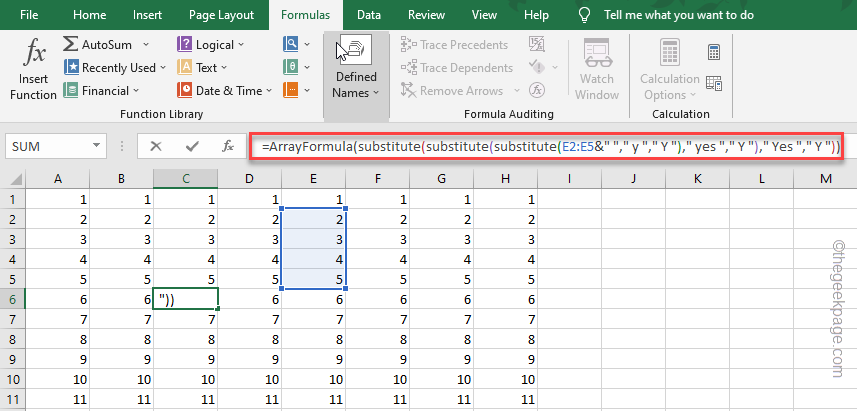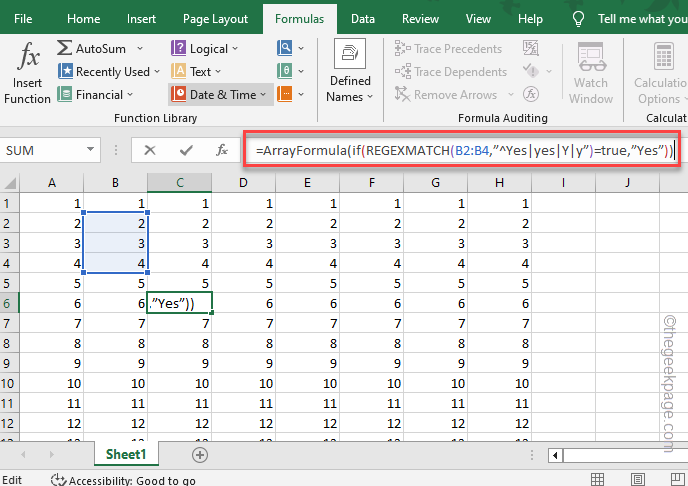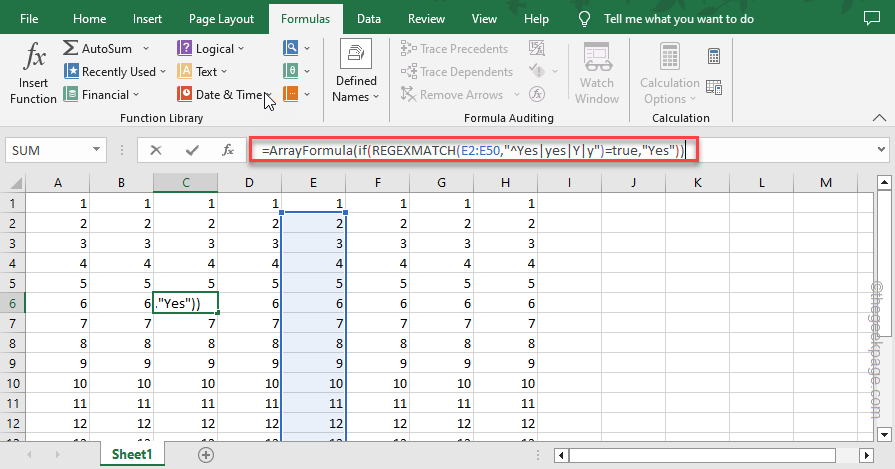# How to Fix An Array value could not be found error in Excel

The “Array value could not be found” error typically appears when Excel tries to access an element of a particular array that is nonexistent. There are several formulae and functions that you may use to troubleshoot this issue. We have described all the possible solutions in this article.

## Fix 1 – Use the Substitute Array formula

Try to bring in the SUBSTITUTE function within the Array function to solve the issue. It is quite easy as you just have to use the ARRAYFOMRULA just before the SUBSTITUTE function. For a better understanding, follow these steps-

Step 1 – Launch Excel and load up the spreadsheet you are working on, if it isn’t opened yet.

Step 2 – Select the cell where you were applying this formula.

Step 3 – Go to the “Formula” bar.

Step 4 – Directly copy-paste this formula, and adjust the cell numbers accordingly.

`=ArrayFormula(substitute(substitute(substitute(E2:E5&" "," y "," Y ")," yes "," Y ")," Yes "," Y "))copied to clipboardcopied to clipboardcopied to clipboardcopied to clipboard`

As you may understand, this will replace the “Y” value with Yes. Change that according to your need.Check if this works. Go for the next formula if this one doesn’t work.

## Fix 2 – Use the RegExMatch formula

RegExMatch is another formula that you may use with the array formula.

Step 1 – Open the Excel spreadsheet.

Step 2 – Go to the “Formula” bar.

Step 3 – Select a cell to apply this formula and paste it directly into the Formula tab.

`=ArrayFormula(if(REGEXMATCH(B2:B4,”^Yes|yes|Y|y”)=true,”Yes”))`

Configure the formula according to your own need.Check if this gets you the desired result.

## Fix 3 – Put both formulae together

In some of the scenarios, you may have to put both formulae together and get the desired output.

Step 1 – After launching the spreadsheet, select a cell that you want to apply the formula on.

Step 2 – After selecting it, head to the “Formula” tab.

Step 3 – Input this formula there in the box.

`=ArrayFormula(if(REGEXMATCH(E2:E50,"^Yes|yes|Y|y")=true,"Yes"))`Arrange the variables according to your need and you should get the result.

## Fix 4 – Using the RegExReplace Formula

The last formula in the line is the RegExReplace Formula. Try using this on your spreadsheet, if everything else has failed so far.

Step 1 – On the Excel spreadsheet, click the “Formula” tab.

Step 2 – Then, paste this in the Formula box. Edit the parameters as you need and run the formula.

```=ArrayFormula(regexreplace(" "&E2:E50&" "," y | yes | Yes "," Y "))

```This way, you won’t face the issue again.

Hopen this helps you resolve the issue.